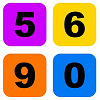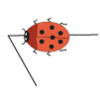Search by Topic

Filter by: Content type:
Age range:
Challenge level:

There are 255 NRICH Mathematical resources connected to Mathematical reasoning & proof, you may find related items under Using, Applying and Reasoning about Mathematics.

Broad Topics > Using, Applying and Reasoning about Mathematics > Mathematical reasoning & proofAlways, Sometimes or Never? Shape

Age 7 to 11 Challenge Level:

Are these statements always true, sometimes true or never true?Always, Sometimes or Never? Number

Age 7 to 11 Challenge Level:

Are these statements always true, sometimes true or never true?Always, Sometimes or Never? KS1

Age 5 to 7 Challenge Level:

Are these statements relating to calculation and properties of shapes always true, sometimes true or never true?Always, Sometimes or Never?

Age 5 to 11 Challenge Level:

Are these statements relating to odd and even numbers always true, sometimes true or never true?Age 5 to 11 Challenge Level:

Who said that adding couldn't be fun?Three Neighbours

Age 7 to 11 Challenge Level:

Look at three 'next door neighbours' amongst the counting numbers. Add them together. What do you notice?Walking Round a Triangle

Age 5 to 7 Challenge Level:

This ladybird is taking a walk round a triangle. Can you see how much he has turned when he gets back to where he started?Square Subtraction

Age 7 to 11 Challenge Level:

Look at what happens when you take a number, square it and subtract your answer. What kind of number do you get? Can you prove it?Take Three Numbers

Age 7 to 11 Challenge Level:

What happens when you add three numbers together? Will your answer be odd or even? How do you know?Odd Times Even

Age 5 to 7 Challenge Level:

This problem looks at how one example of your choice can show something about the general structure of multiplication.Two Numbers Under the Microscope

Age 5 to 7 Challenge Level:

This investigates one particular property of number by looking closely at an example of adding two odd numbers together.Advent Calendar 2011 - Secondary

Age 11 to 18 Challenge Level:

Advent Calendar 2011 - a mathematical activity for each day during the run-up to Christmas.Tower of Hanoi

Age 11 to 14 Challenge Level:

The Tower of Hanoi is an ancient mathematical challenge. Working on the building blocks may help you to explain the patterns you notice.Age 11 to 16 Challenge Level:

Draw some quadrilaterals on a 9-point circle and work out the angles. Is there a theorem?Pythagoras Proofs

Age 14 to 16 Challenge Level:

Can you make sense of these three proofs of Pythagoras' Theorem?Age 11 to 14 Challenge Level:

Powers of numbers behave in surprising ways. Take a look at some of these and try to explain why they are true.Elevenses

Age 11 to 14 Challenge Level:

How many pairs of numbers can you find that add up to a multiple of 11? Do you notice anything interesting about your results?Direct Logic

Age 16 to 18 Challenge Level:

Can you work through these direct proofs, using our interactive proof sorters?Contrary Logic

Age 16 to 18 Challenge Level:

Can you invert the logic to prove these statements?Iffy Logic

Age 14 to 18 Challenge Level:

Can you rearrange the cards to make a series of correct mathematical statements?What Do You Need?

Age 7 to 11 Challenge Level:

Four of these clues are needed to find the chosen number on this grid and four are true but do nothing to help in finding the number. Can you sort out the clues and find the number?Multiplication Square

Age 14 to 16 Challenge Level:

Pick a square within a multiplication square and add the numbers on each diagonal. What do you notice?Salinon

Age 14 to 16 Challenge Level:

This shape comprises four semi-circles. What is the relationship between the area of the shaded region and the area of the circle on AB as diameter?Seven Squares - Group-worthy Task

Age 11 to 14 Challenge Level:

Choose a couple of the sequences. Try to picture how to make the next, and the next, and the next... Can you describe your reasoning?Perfectly Square

Age 14 to 16 Challenge Level:

The sums of the squares of three related numbers is also a perfect square - can you explain why?More Number Pyramids

Age 11 to 14 Challenge Level:

When number pyramids have a sequence on the bottom layer, some interesting patterns emerge...Always Perfect

Age 14 to 16 Challenge Level:

Show that if you add 1 to the product of four consecutive numbers the answer is ALWAYS a perfect square.Children at Large

Age 11 to 14 Challenge Level:

There are four children in a family, two girls, Kate and Sally, and two boys, Tom and Ben. How old are the children?For What?

Age 14 to 16 Challenge Level:

Prove that if the integer n is divisible by 4 then it can be written as the difference of two squares.Leonardo's Problem

Age 14 to 18 Challenge Level:

A, B & C own a half, a third and a sixth of a coin collection. Each grab some coins, return some, then share equally what they had put back, finishing with their own share. How rich are they?Take Three from Five

Age 14 to 16 Challenge Level:

Caroline and James pick sets of five numbers. Charlie chooses three of them that add together to make a multiple of three. Can they stop him?Age 16 to 18 Challenge Level:

Find all real solutions of the equation (x^2-7x+11)^(x^2-11x+30) = 1.Largest Product

Age 11 to 14 Challenge Level:

Which set of numbers that add to 10 have the largest product?Proof Sorter - Sum of an AP

Age 16 to 18 Challenge Level:

Use this interactivity to sort out the steps of the proof of the formula for the sum of an arithmetic series. The 'thermometer' will tell you how you are doingProof Sorter - Quadratic Equation

Age 14 to 18 Challenge Level:

This is an interactivity in which you have to sort the steps in the completion of the square into the correct order to prove the formula for the solutions of quadratic equations.Proofs with Pictures

Age 14 to 18

Some diagrammatic 'proofs' of algebraic identities and inequalities.Telescoping Functions

Age 16 to 18

Take a complicated fraction with the product of five quartics top and bottom and reduce this to a whole number. This is a numerical example involving some clever algebra.1 Step 2 Step

Age 11 to 14 Challenge Level:

Liam's house has a staircase with 12 steps. He can go down the steps one at a time or two at time. In how many different ways can Liam go down the 12 steps?Number Rules - OK

Age 14 to 16 Challenge Level:

Can you convince me of each of the following: If a square number is multiplied by a square number the product is ALWAYS a square number...Lens Angle

Age 14 to 16 Challenge Level:

Find the missing angle between the two secants to the circle when the two angles at the centre subtended by the arcs created by the intersections of the secants and the circle are 50 and 120 degrees.Iff

Age 14 to 18 Challenge Level:

Take a triangular number, multiply it by 8 and add 1. What is special about your answer? Can you prove it?Why 24?

Age 14 to 16 Challenge Level:

Take any prime number greater than 3 , square it and subtract one. Working on the building blocks will help you to explain what is special about your results.Angle Trisection

Age 14 to 16 Challenge Level:

It is impossible to trisect an angle using only ruler and compasses but it can be done using a carpenter's square.Zig Zag

Age 14 to 16 Challenge Level:

Four identical right angled triangles are drawn on the sides of a square. Two face out, two face in. Why do the four vertices marked with dots lie on one line?Unit Interval

Age 14 to 18 Challenge Level:

Take any two numbers between 0 and 1. Prove that the sum of the numbers is always less than one plus their product?Age 16 to 18 Challenge Level:

Find all positive integers a and b for which the two equations: x^2-ax+b = 0 and x^2-bx+a = 0 both have positive integer solutions.Mechanical Integration

Age 16 to 18 Challenge Level:

To find the integral of a polynomial, evaluate it at some special points and add multiples of these values.Prime AP

Age 16 to 18 Challenge Level:

What can you say about the common difference of an AP where every term is prime?Common Divisor

Age 14 to 16 Challenge Level:

Find the largest integer which divides every member of the following sequence: 1^5-1, 2^5-2, 3^5-3, ... n^5-n.There's a Limit

Age 14 to 18 Challenge Level:

Explore the continued fraction: 2+3/(2+3/(2+3/2+...)) What do you notice when successive terms are taken? What happens to the terms if the fraction goes on indefinitely?